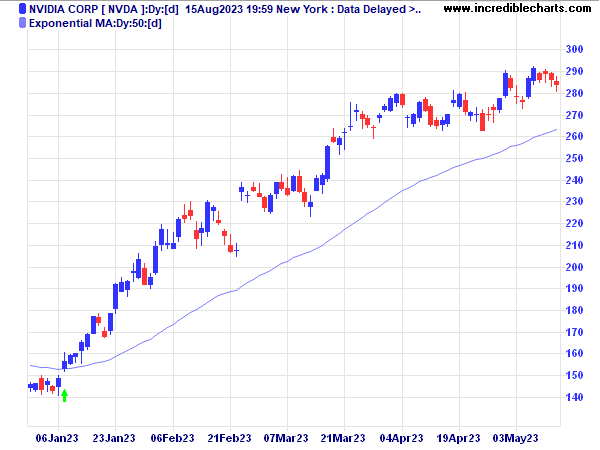# Moving Average Indicator

Moving averages provide an objective measure of trend direction by smoothing price data. Normally calculated using closing prices, the moving average can also be used with median, typical , weighted closing, and high, low or open prices as well as other indicators.## Moving Average Time Frames

Shorter length moving averages are more sensitive and identify new trends earlier, but also give more false alarms. Longer moving averages are more reliable but less responsive, only picking up the big trends.

Use a moving average that is half the length of the cycle that you are tracking. If the peak-to-peak cycle length is roughly 30 days, then a 15 day moving average is appropriate. If 20 days, then a 10 day moving average is appropriate. Some traders, however, will use 14 and 9 day moving averages for the above cycles in the hope of generating signals slightly ahead of the market. Others favor the Fibonacci numbers of 5, 8, 13 and 21.

• 100 to 200 Day (20 to 40 Week) moving averages are popular for longer cycles;
• 20 to 65 Day ( 4 to 13 Week) moving averages are useful for intermediate cycles; and
• 5 to 20 Days for short cycles.

The simplest moving average system generates signals when price crosses the moving average:

• Go long when price crosses to above the moving average from below.
• Go short when price crosses to below the moving average from above.

The system is prone to whipsaws in ranging markets, with price crossing back and forth across the moving average, generating a large number of false signals. For that reason, moving average systems normally employ filters to reduce whipsaws.

More sophisticated systems use more than one moving average.

• Two Moving Averages uses a faster moving average as a substitute for closing price.
• Three Moving Averages employs a the third moving average to identify when price is ranging.
• Multiple Moving Averages use a series of six fast moving averages and six slow moving averages to confirm each other.

• Displaced Moving Averages are useful for trend-following purposes, reducing the number of whipsaws.
• Keltner Channels use bands plotted at a multiple of average true range to filter moving average crossovers.
• The popular MACD ("Moving Average Convergence Divergence") indicator is a variation of the two moving average system, plotted as an oscillator which subtracts the slow moving average from the fast moving average.

## Moving Average Types

There are several different types of moving averages, each with their own peculiarities.

• Simple moving averages are the easiest to construct, but also the most prone to distortion.
• Weighted moving averages are difficult to construct, but reliable.
• Exponential moving averages achieve the benefits of weighting combined with ease of construction.
• Wilder moving averages are used mainly in indicators developed by J. Welles Wilder. Essentially the same formula as exponential moving averages, they use different weightings — for which users need to make allowance.

## Setup

Indicator Panel shows how to set up moving averages.

The default setting is a 21 day exponential moving average.